AC Circuits Ohm’s Law for AC Circuits The rules and equations for DC circuits apply to AC circuits only when the circuits contain resistance alone, as in the case of lamps and heating elements. In order to use effective values of voltage and current in AC circuits, the effect of inductance and capacitance with resistance must be considered. The combined effects of resistance, inductive reactance, and capacitive reactance make up the total opposition to current flow in an AC circuit. This total opposition is called impedance and is represented by the letter Z. The unit for the measurement of impedance is the ohm. Series AC Circuits If an AC circuit consists of resistance only, the value of the impedance is the same as the resistance, and Ohm’s law for an AC circuit, I = E/Z, is exactly the same as for a DC circuit. In Figure 10-126 a series circuit containing a lamp with 11 ohms resistance connected across a source is illustrated.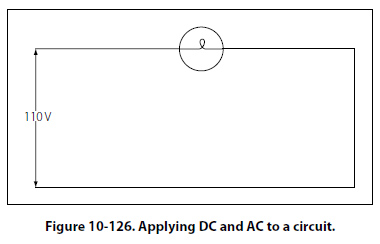To find how much current will flow if 110 volts DC is applied and how much current will flow if 110 volts AC are applied, the following examples are solved: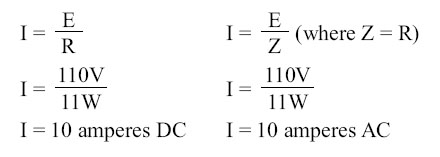When AC circuits contain resistance and either inductance or capacitance, the impedance, Z, is not the same as the resistance, R. The impedance of a circuit is the circuit’s total opposition to the flow of current. In an AC circuit, this opposition consists of resistance and reactance, either inductive or capacitive or elements of both. Resistance and reactance cannot be added directly, but they can be considered as two forces acting at right angles to each other. Thus, the relation between resistance, reactance, and impedance may be illustrated by a right triangle. [Figure 10-127]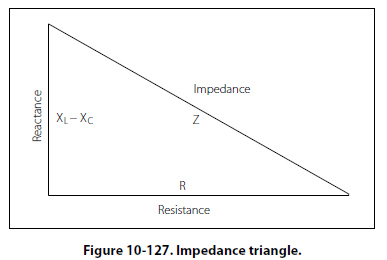Since these quantities may be related to the sides of a right triangle, the formula for finding the impedance, or total opposition to current flow in an AC circuit, can be found by using the law of right triangles. This theorem, called the Pythagorean theorem, applies to any right triangle. It states that the square of the hypotenuse is equal to the sum of the squares of the other two sides. Thus, the value of any side of a right triangle can be found if the other two sides are known. If an AC circuit contains resistance and inductance, as shown in Figure 10-128, the relation between the sides can be stated as: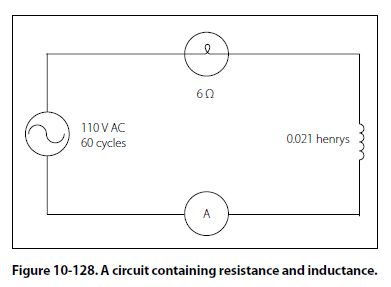Z2 = R2 + XL2 The square root of both sides of the equation gives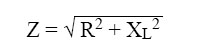This formula can be used to determine the impedance when the values of inductive reactance and resistance are known. It can be modified to solve for impedance in circuits containing capacitive reactance and resistance by substituting XC in the formula in place of XL. In circuits containing resistance with both inductive and capacitive reactance, the reactances can be combined, but because their effects in the circuit are exactly opposite, they are combined by subtraction: X = XL - XC or X = XC - XL (the smaller number is always subtracted from the larger). In Figure 10-128, a series circuit consisting of resistance and inductance connected in series is connected to a source of 110 volts at 60 cycles per second. The resistive element is a lamp with 6 ohms resistance, and the inductive element is a coil with an inductance of 0.021 henry. What is the value of the impedance and the current through the lamp and the coil?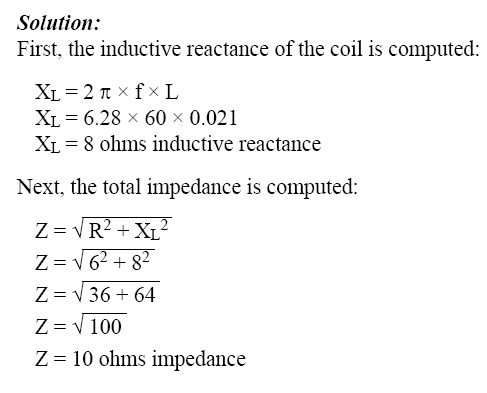Then the current flow,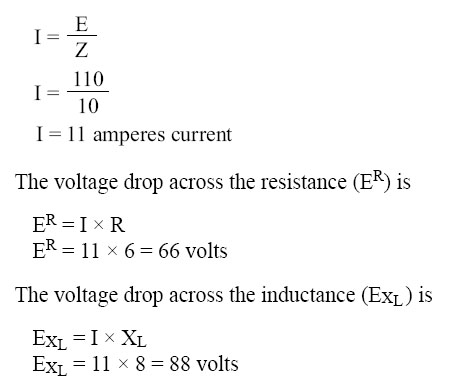The sum of the two voltages is greater than the impressed voltage. This results from the fact that the two voltages are out of phase and, as such, represent the maximum voltage. If the voltage in the circuit is measured by a voltmeter, it will be approximately 110 volts, the impressed voltage. This can be proved by the equation,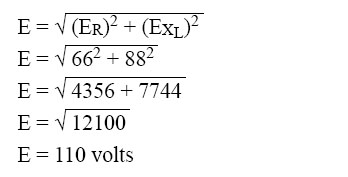In Figure 10-129, a series circuit is illustrated in which a capacitor of 200 µf is connected in series with a 10 ohm lamp.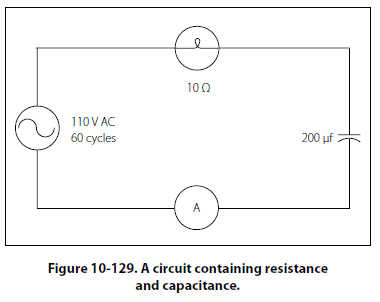What is the value of the impedance, the current flow, and the voltage drop across the lamp?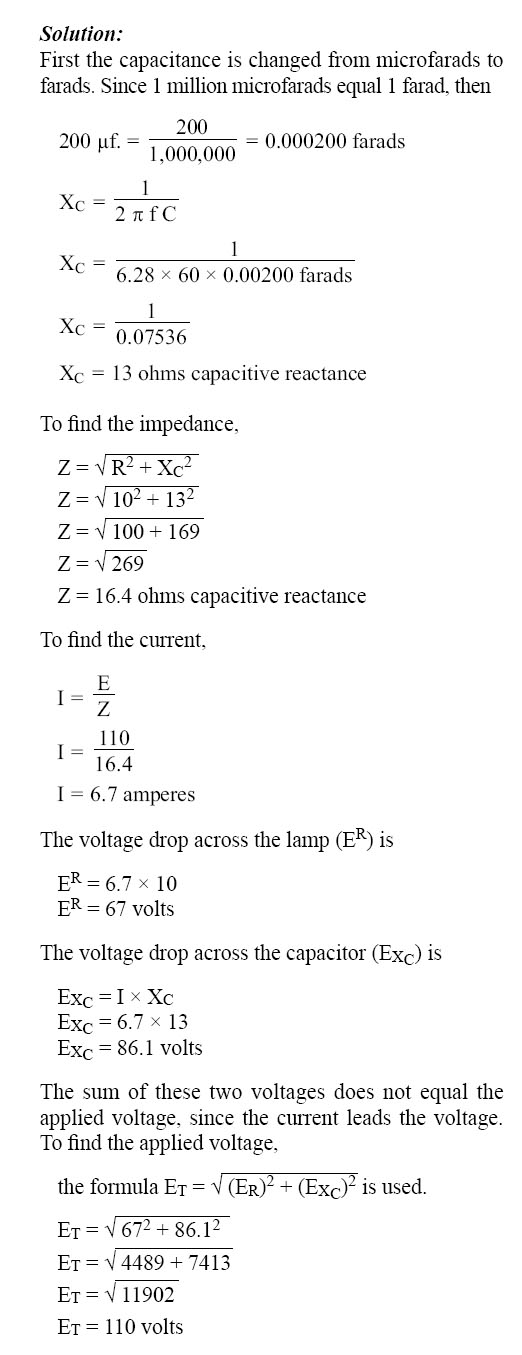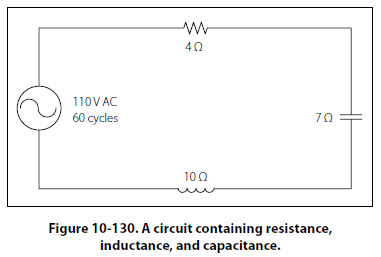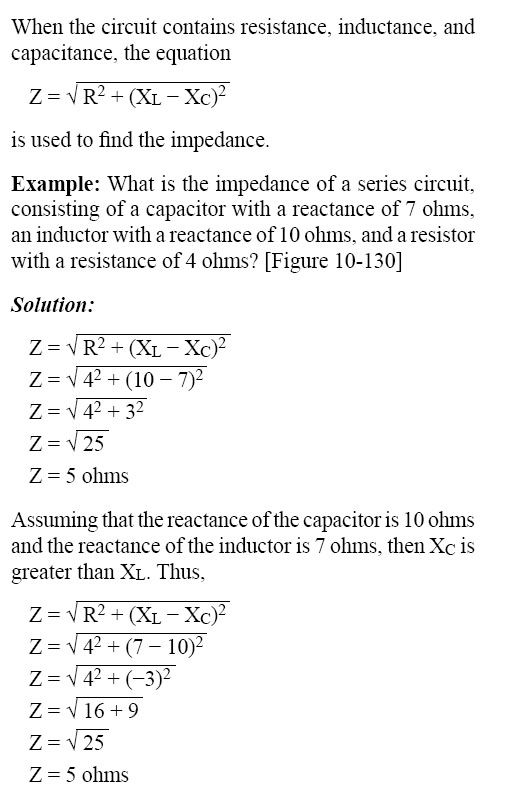©AvStop Online Magazine                                                                                                                                                      Contact Us              Return To Books